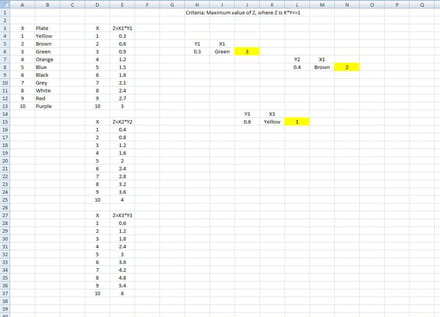# VBA Tricky Question

Closed
marsdjinn Posts 1 Registration date Friday March 4, 2016 Status Member Last seen March 4, 2016 - Mar 4, 2016 at 09:28 PM
TrowaD Posts 2921 Registration date Sunday September 12, 2010 Status Moderator Last seen January 16, 2023 - Mar 8, 2016 at 11:53 AM
Hi All,

I am working on excel and I need some help with VBA/macros.

Basically, I have a variable called Y and I have a table of set values, called X which are vlookup valves, ranging from 1-10. The X values correspond to different colours 1.e x1=blue x2=red etc.

X Colour
1 Red
2 Blue
3 Green
4 Purple
etc

The equation Z where Z=X*Y and must meet the criteria where X*Y<=1

For example, if Y=0.3 the value should return is X=3 since the Z value is the max value where 0.9 which is <=1

X Z=X*Y
1 1*0.3=0.3
2 2*0.3=0.6
3 3*0.3=0.9
4 4*0.3=1.2
etc

The value is then used to lookup from a table to produce the colour i.e. X=3 therefore show the text green in another cell.

However, the variable Y needs to be copied into other places in the workbook

I.e. one place might have Y as 0.6, whereas another place may have Y was 0.4

I was wondering if it would be possible to therefore embed a formula into any location and run it so that the formula will use the same X values list from 1-10, but vary to Y value to recalculate calculate Z until the criteria is met (X*Y<=1)?I hope this makes sense, any help is much appreciated!

Kind regards,
Max